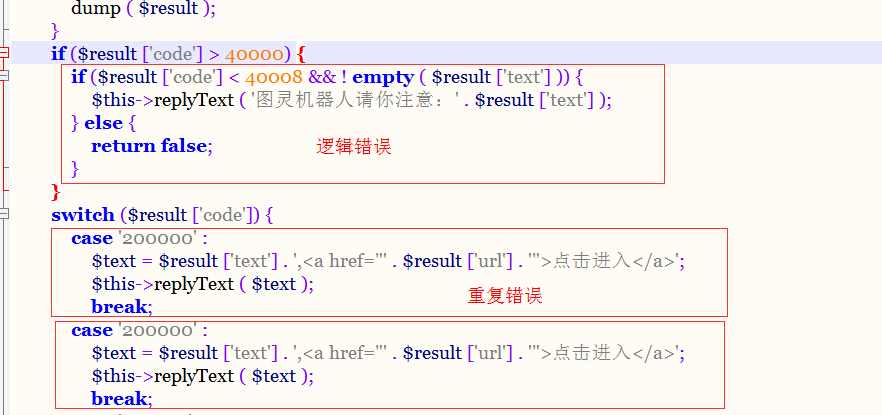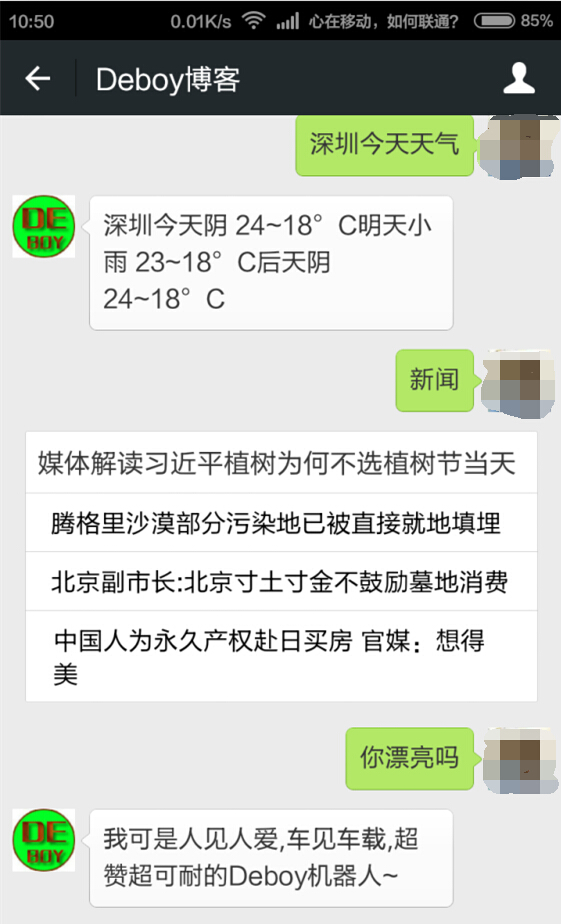`http://www.tuling123.com/openapi/api?key=这里填写你的KEY=讲个笑话`

`{“code”:100000,”text”:”父亲：“我亲爱的孩子，如果你想找个称心如意的丈夫，那你就和隔壁邻居的儿子结婚吧，他真心地爱你。”<br>女儿：“爸爸，您怎么知道？”<br>父亲：“我借了他许多钱，已达六个月之久，但他没有来要。”<br>”}`

weiphp2.0中的原版代码如下

`// 图灵机器人    private function _tuling(\$keyword) {        \$api_url = \$this->config [‘tuling_url’] . “?key=” . \$this->config [‘tuling_key’] . “&info=” . \$keyword;`

``````\$result = file_get_contents ( \$api_url );
\$result = json_decode ( \$result, true );
if (\$_GET ['format'] == 'test') {
dump ( '图灵机器人结果：' );
dump ( \$result );
}
if (\$result ['code'] &gt; 40000) {
if (\$result ['code'] &lt; 40008 &amp;&amp; ! empty ( \$result ['text'] )) {
\$this-&gt;replyText ( '图灵机器人请你注意：' . \$result ['text'] );
} else {
return false;
}
}
switch (\$result ['code']) {
case '200000' :
\$text = \$result ['text'] . ',&lt;a href="' . \$result ['url'] . '"&gt;点击进入&lt;/a&gt;';
break;
case '200000' :
\$text = \$result ['text'] . ',&lt;a href="' . \$result ['url'] . '"&gt;点击进入&lt;/a&gt;';
break;</pre>
```````        if (\$result [‘code’] > 40000) {            if (\$result [‘code’] < 40008 && ! empty ( \$result [‘text’] )) {                \$this->replyText ( ‘图灵机器人请你注意：’ . \$result [‘text’] );            }        }        switch (\$result [‘code’]) {            case ‘200000’ :                \$text = \$result [‘text’] . ‘,<a href=”‘ . \$result [‘url’] . ‘“>点击进入</a>’;                \$this->replyText ( \$text );                break;`